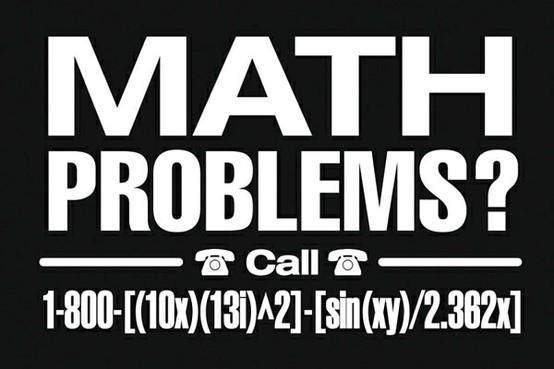Welcome, Guest

###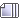Author Topic: All things mathematical.  (Read 13394 times)

0 Members and 1 Guest are viewing this topic.

####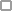The Lurker

• Grendel's Mom
•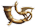•• Posts: 9750
• Liked: 5535##### Re: All things mathematical.
« Reply #105 on: August 15, 2013, 07:34:10 AM »
The guy reminds me of Pat Kilbane from MadTv.

####Thrifty Version II

• Big Montana
•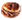•• Posts: 984
• Liked: 556
• Now with 30% Less Fat!##### Re: All things mathematical.
« Reply #106 on: September 06, 2013, 06:44:45 PM »
Okay, I'm trying to solve this one so that I can finish a programming assignment.  I don't know if there's a solution given the information that I have.  If not, I'll need to find some other way to complete the program.

Okay, so imagine a standard 5 pointed star like this:The star is inside a circle.  If you know the Cartesian coordinates of all the 10 points of the star (the 10 pointy ends as well as the 5 exterior angles), can you approximate the diameter of the circle?  And can you find the center of the circle such that the whole star is within it?  The circle has to envelop the star; it doesn't have to be perfectly tight.

The coordinates of the star are (55,0), (67,36), (109,36), (73,54), (83,96), (55,72), (27,96), (37,54), (1,36), and (43,36).I mean it looks like you can get the diameter by taking the distance from (1,36) to (110,36).  You get a circle that should be big enough.  But I guess my other question then, is.... how do you find the center of the star?

I'm wondering if there's something I'm not seeing or if there's just not enough information to solve this.

####MartyS (Gromit)##### Re: All things mathematical.
« Reply #107 on: September 06, 2013, 07:38:03 PM »
Since it's not a regular star (irregular polygon)there is no defined center.
If you draw lines between the tips of the star you have a 5 sided irregular polygon (can't call it a pentagon since the sides are not equal).  I think you could find the centroid (center of mass) of the polygon, then find the farthest point on the star away from the centroid, use those 2 values for the center and radius of the circle and I think that would do it...?

####McDonald's##### Re: All things mathematical.
« Reply #108 on: September 06, 2013, 09:37:35 PM »
use two points on the outside of the circle, get a slope.  use that and a point to find an intercept.  voila equation of line.  use negative inverse of the slope for orthogonal.  intersects the point on the far side.  boom equation of a line crossing through center of star.  now do it all over again for two different points on the circle, find the intercept in the center.  find distance from intercept to outside point.  double it.  BOOM EIGHT SECONDS

####Thrifty Version II

• Big Montana
••• Posts: 984
• Liked: 556
• Now with 30% Less Fat!##### Re: All things mathematical.
« Reply #109 on: September 07, 2013, 04:55:54 AM »
Since it's not a regular star (irregular polygon)there is no defined center.
If you draw lines between the tips of the star you have a 5 sided irregular polygon (can't call it a pentagon since the sides are not equal).  I think you could find the centroid (center of mass) of the polygon, then find the farthest point on the star away from the centroid, use those 2 values for the center and radius of the circle and I think that would do it...?

I had to look up the term "centroid" and find out how to calculate it.  You sum the x-coordinates and divide by the number of points to find the x-coordinate of the centroid?  Then do the same with the y-coordinates for the y of the centroid?  Right?  I did that, got 551 and 516, respectively, as the x and y sums.  Divide by 10 and I get (55.1, 51.6).  Rounded those off to (55,52), and add that to the plot, and I got something that looks good enough.  Now to plug that into my program and see if it works out.

####goflyblind

• Ephialtes
•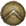•• Posts: 7963
• Liked: 7357
• i was told there would be sugar syrup.##### Re: All things mathematical.
« Reply #110 on: September 07, 2013, 06:08:38 AM »
since the {(1,36),(43,36)} and {(67,36),(110,36)} segments aren't the same length, using d = 110-1 = 109 won't work. to be rigorous, you'd want to find the length of the vector between each of the points and the centroid, then pick the longest one as your radius.

i.e.
r_i = (Abs[Cx - x_i], Abs[Cy - y_i])
r = Max[Sqrt[r_i.r_i]]
dF = 0
d*F = J

####Thrifty Version II

• Big Montana
••• Posts: 984
• Liked: 556
• Now with 30% Less Fat!##### Re: All things mathematical.
« Reply #111 on: September 07, 2013, 10:39:17 AM »
I stumbled into a working solution, and I'm not really sure how.  I forgot that in Java, to draw a circle, you draw either an ellipse (with equal length and width) or an arc of 360 degrees.  You give it x, y, width, and length for the ellipse.  Except x and y aren't the center of the ellipse.  They're the location of the upper left corner of an invisible box that contains the ellipse.  So I had to find the approximate center of the star and then do this:

Shape myCircle = new Ellipse2D.Double(centerX-(diameter/2), centerY-(diameter/2), diameter, diameter);

Where the center of the star is at (centerX, centerY).

Which gets me this:Which is good enough, but I'm disturbed that I stumbled into it.

####RVR II

• Global Moderator
•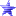•• Posts: 56120
• Liked: 5001
• There can be Only 1...##### Re: All things mathematical.
« Reply #112 on: September 07, 2013, 12:18:18 PM »
Saw this and thought it was funny